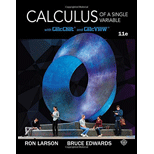Chapter 2.1, Problem 95E### Calculus of a Single Variable

11th Edition
Ron Larson + 1 other
ISBN: 9781337275361

#### Solutions

Chapter
Section### Calculus of a Single Variable

11th Edition
Ron Larson + 1 other
ISBN: 9781337275361
Textbook Problem

# True or False? In Exercises 93-96, determine whether the statement is true or false. If it is false, explain why or give an example that shows it is false.If a function has derivatives from both the right and the left at a point, then it is differentiable at that point.

To determine

To calculate: Whether the given statement “If a function has derivatives from both the right and left at a point, then it is differentiable at that point” is true or false.

Explanation

Given: If a function has derivatives from both the right and left at a point, then it is differentiable at that point.

It is not necessary for a function to be differentiable if it has derivatives from both left-and right-hand sides of a point. In fact, for a function to be differentiable, the function should not only have left- and right-hand derivatives, but they should also be equal.

One such example is that of the function f(x)=|x| at x=0

### Still sussing out bartleby?

Check out a sample textbook solution.

See a sample solution

#### The Solution to Your Study Problems

Bartleby provides explanations to thousands of textbook problems written by our experts, many with advanced degrees!

Get Started

## Additional Math Solutions

#### Find more solutions based on key concepts